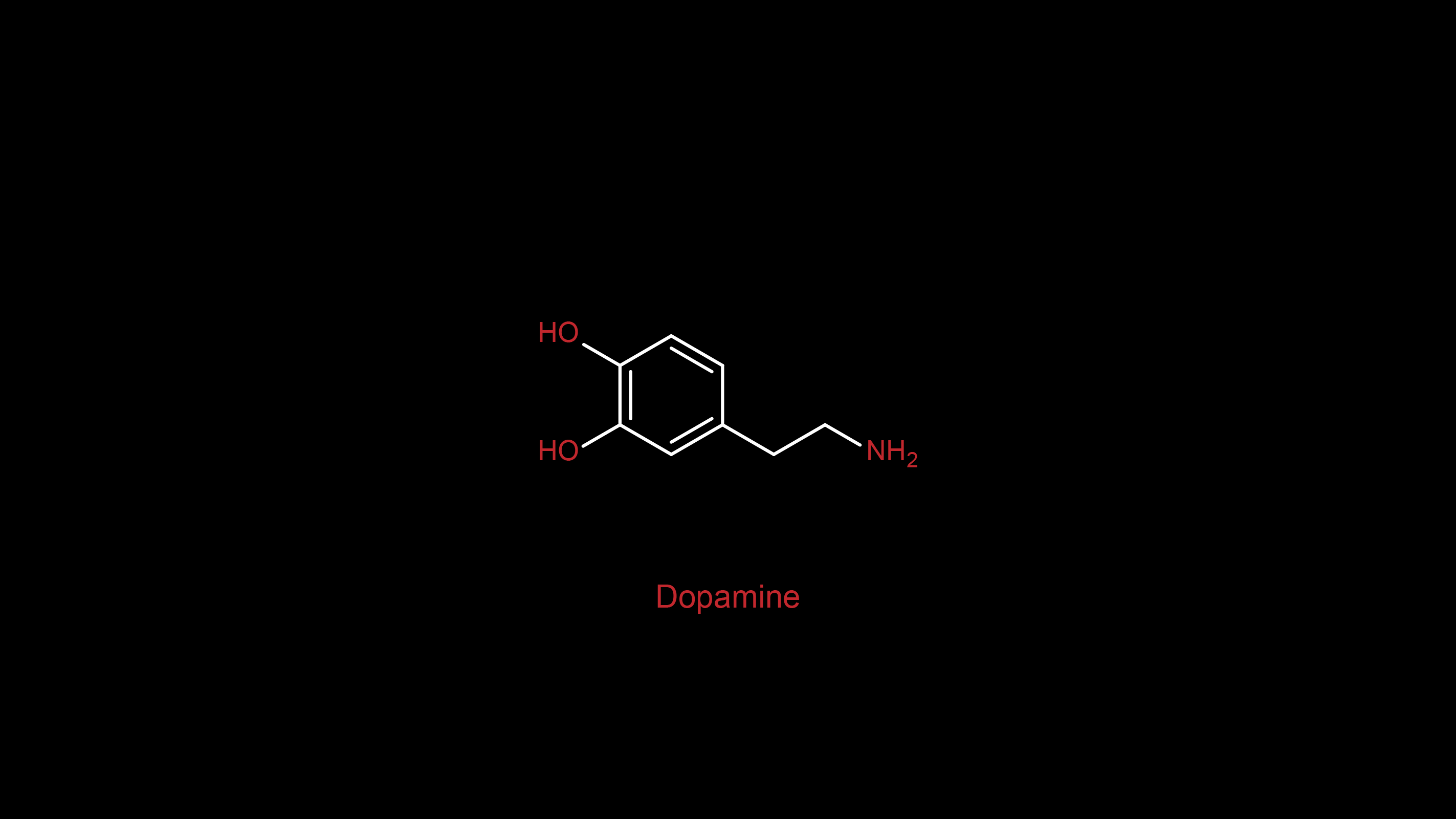# 4.09广搜题解

## 林大超市买水果

### Sample Input

``````20 5 3
1 2 3 15 6``````

### Sample Output

``Yes``

### 题解``````//买这件水果
todo.push({cur+1,number+1,money-price[cur]});``````

``````//不买这件水果
todo.push({cur+1,number,money});``````

``if (money==0&&number==want) return 1;``

### 综上，有AC代码：

``````/*
* @Author: Mr.Sen
* @LastEditTime: 2020-04-09 21:08:23
* @Website1: https://449293786.site
* @原创代码，版权所有，转载请注明原作者
*/
#include<bits/stdc++.h>
using namespace std;
struct node
{
int cur;
//当前查找的商品
int number;
//已经购买的商品
int money;
//还剩的钱
};
queue <node> todo;
int price,want,all;
int bfs(int money)
{
todo.push({0,0,money});
while (!todo.empty())
{
node tmp=todo.front();
todo.pop();
int cur=tmp.cur;
int number=tmp.number;
int money=tmp.money;
//读取每个节点的状态
if (money==0&&number==want) return 1;
//如果钱刚好花完并且买足了了自己想要的商品，就返回真
if (money-price[cur]>=0&&number<want&&cur<all)
{
todo.push({cur+1,number+1,money-price[cur]});
//买，则钱变少，数量变多
todo.push({cur+1,number,money});
//不买，钱不变，数量不变，跳过当前商品
}
}
return 0;
}
int main()
{
int money;
cin>>money>>all>>want;
for (int i=0;i<all;i++)
scanf("%d",&price[i]);
bfs(money)==1?printf("Yes\n"):printf("No\n");
return 0;
}``````

## 最大黑色区域-搜索

### Sample Input

``````5 6
0 1 1 0 0 1
1 1 0 1 0 1
0 1 0 0 1 0
0 0 0 1 1 1
1 0 1 1 1 0``````

### Sample Output

``7``

### AC代码：

``````/*
* @Author: Mr.Sen
* @LastEditTime: 2020-04-05 22:43:42
* @Website1: 449293786.site
*/

#include<bits/stdc++.h>
using namespace std;

struct node {
int x;
int y;
};

queue<node>todo;
queue<node>bg;
int n,m;
int x2={1,-1,0,0};
int y2={0,0,1,-1};
int maps;
int walked;
//creat a map
int bfs(int bx,int by){
int ans=0;
maps[bx][by]=0;
todo.push({bx,by});
while(!todo.empty()){
node tmp;
tmp=todo.front();
todo.pop();
ans++;
int x3=tmp.x;
int y3=tmp.y;
// cout<<x3<<" "<<y3<<endl;
for (int i=0;i<4;i++){
int x=x2[i]+x3;
int y=y2[i]+y3;
if (x>=0&&x<n&&y>=0&&y<m&&maps[x][y]==1){
todo.push({x,y});
maps[x][y]=0;
}
}
}
return ans;
}
int main(){
int ans=0;
int bx,by;
cin>>n>>m;
for(int i=0;i<n;i++){
for (int j=0;j<m;j++){
scanf("%d",&maps[i][j]);
if (maps[i][j]){
bg.push({i,j});
}
}
}
while(!bg.empty()){
node tmp=bg.front();
bg.pop();
if (maps[tmp.x][tmp.y]){
int res=bfs(tmp.x,tmp.y);
ans=max(res,ans);
}
}
cout<<ans<<endl;
return 0;
}``````